# Subtraction of four digit number | Maths Grade 4

Learn about Subtraction of four digit numbers, 4 digit subtraction without borrowing, 4 digit subtraction with borrowing

### Subtraction of four digit number without borrowing

Question 1

Subtract the following: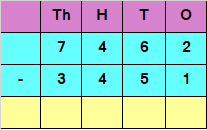Explanation

Subtracting the digits at ones place
2 – 1 = 1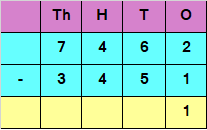Subtracting the digits at tens place
6 – 5 = 1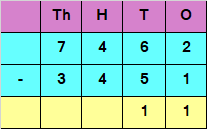Subtracting the digits at hundreds place
4 – 4 = 0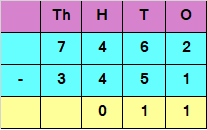Subtracting the digits at thousands place
7 – 3 = 4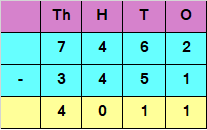Hence, the difference is 4011

Question 2

Subtract the following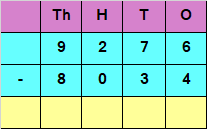Explanation

Subtracting the digits at ones place
6 – 4 = 2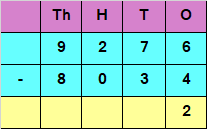Subtracting the digits at tens place
7 – 3 = 4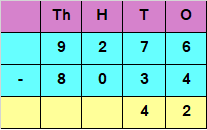Subtracting the digits at hundreds place
2 – 0 = 2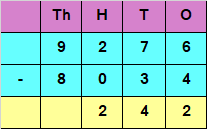Subtracting the digits at thousands place
9 – 8 = 1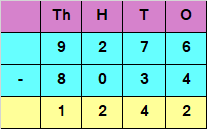Hence, the difference is 1242

### Subtraction of four digit number with borrowing

Question 1

Subtract the following: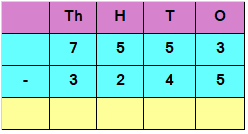Explanation

If we want to Subtract the digits at ones place, we have
3 – 5
But 5 is greater than 3
We would need to borrow 1 from 5 in tens place
3 in ones place would now become 13 and 5 in tens place would be reduced to 4
So, 13 – 5 = 8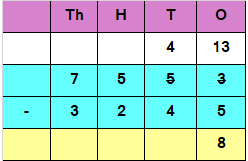Subtracting the digits at tens place
4 – 4 = 0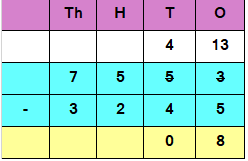Subtracting the digits at hundreds place
5 – 2 = 3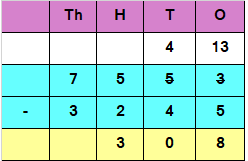Subtracting the digits at thousands place
7 – 3 = 4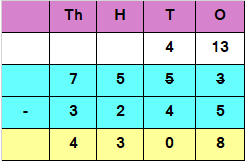Hence, the difference is 4308

Question 2

Subtract the following: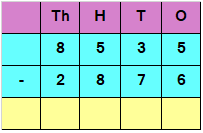Explanation

If we want to Subtract the digits at ones place, we have
5 – 6
But 6 is greater than 5
We would need to borrow 1 from 3 in tens place
5 in ones place would now become 15 and 3 in tens place would be reduced to 2
So, 15 – 6 = 9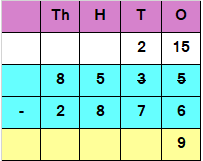If we want to Subtract the digits at tens place, we have
2 – 7
But 7 is greater than 2
We would need to borrow 1 from 5 in hundred place
2 in tens place would now become 12 and 5 in hundred place would be reduced to 4
So, 12 – 7 = 5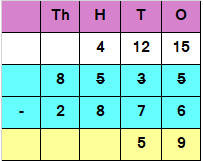If we want to Subtract the digits at hundred place, we have
4 – 8
But 8 is greater than 4
We would need to borrow 1 from 8 in thousand place
4 in hundred place would now become 14 and 8 in thousand place would be reduced to 7
So, 14 – 8 = 6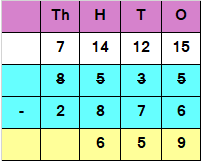Subtracting the digits at thousands place
7 – 2 = 5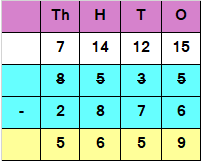Hence, the difference is 5659